﻿ Frequency Domain Comparison of Multi-lump and Distributed Parameter Models for Pressurized Water Reactor Cores

### Frequency Domain Comparison of Multi-lump and Distributed Parameter Models for Pressurized Water Rea...

Taipeng Zhang, Keith E. HolbertOPEN ACCESSPEER-REVIEWED

## Frequency Domain Comparison of Multi-lump and Distributed Parameter Models for Pressurized Water Reactor Cores

Taipeng Zhang1,, Keith E. Holbert1

1School of Electrical, Computer and Energy Engineering, Arizona State University, Tempe, USA

### Abstract

Multi-lump and distributed parameter models are used to analyze the frequency behavior of a pressurized water reactor (PWR). The distributed parameter model is built upon the partial differential equations describing heat transfer and fluid flow in the reactor core. For comparison, a lumped parameter reactor core model with multiple fuel and coolant lumps is employed. The features of the transfer functions for both models are evaluated. The distributed parameter model has the ability to offer an accurate transfer function at any location throughout the reactor core. In contrast, the multi-lump parameter model only provides an average value in a given region (lump). Comparisons show that the multi-lump model results are only most favorable for frequencies less than ~0.1 Hz.

### At a glance: Figures

12345
Prev Next

• Zhang, Taipeng, and Keith E. Holbert. "Frequency Domain Comparison of Multi-lump and Distributed Parameter Models for Pressurized Water Reactor Cores." American Journal of Energy Research 1.1 (2013): 17-24.
• Zhang, T. , & Holbert, K. E. (2013). Frequency Domain Comparison of Multi-lump and Distributed Parameter Models for Pressurized Water Reactor Cores. American Journal of Energy Research, 1(1), 17-24.
• Zhang, Taipeng, and Keith E. Holbert. "Frequency Domain Comparison of Multi-lump and Distributed Parameter Models for Pressurized Water Reactor Cores." American Journal of Energy Research 1, no. 1 (2013): 17-24.

 Import into BibTeX Import into EndNote Import into RefMan Import into RefWorks

### 1. Introduction

This investigation compares and contrasts distributed and lumped parameter models for pressurized water reactor (PWR) cores. The distributed and multi-lump representations are built upon partial and ordinary differential equations, respectively. These models are utilized in this study to analyze the frequency domain behavior of a nuclear power reactor.

Previous researchers have developed methods based on different multi-lump parameter models to evaluate the behavior of the nuclear reactor core; for example, see , . However, the multi-lump approach is based on average theory; it is difficult to arrive at an accurate result at any exact point under study. To improve model resolution, the number of lumps could be increased to a large number. But this makes an analytical solution difficult and a numerical solution may require excessive computer time. The limitations of the lumped parameter technique is an impetus to construct a distributed-parameter model so that wherever the location of interest, the actual state variable values at that position can be calculated. With the distributed-parameter representation, the transfer function between any input and output at any location throughout the reactor core can be derived, plotted and analyzed.

Early distributed parameter models were developed by Gyftopoulos and Smets, who performed a one-dimensional slab geometry analysis in which the fuel, moderator and coolant were three separate slabs . Some researchers were motivated to pursue such representations due to work with boiling water reactors, whose modeling requires consideration of a moving boundary between single and two phase coolant flow. For instance, Ciechanowicz developed such models [4, 5, 6].

Although the distributed parameter model is more accurate than the multi-lump approach, the precision of a distributed parameter model is not always necessary, and in such situations, lumped parameter models may suffice. This need was a motivation for this research to determine when one model is more appropriate than the other.

While distributed parameter models for reactor cores are not new, the computational tools available to researchers and practioners have advanced dramatically. Original distributed parameter models (circa 1960) were carried out using analog computers. Besides, the reactor power levels have grown significantly since that work was performed. Furthermore, reactor monitoring and diagnostic methods using noise analysis often employ frequency domain models of phenomena. For an extensive treatise on the subject of power reactor noise analysis, see .

Reactor core modeling using both multi-lump and distributed parameter representations is first presented in Section 2 of this paper. Afterward, detailed comparisons of the transfer functions obtained from the two models are made using data from a large commercial nuclear power station. The differences between the models are evaluated and explained. These comparisons provide a deeper understanding of the behavior of the reactor core as well as revealing the advantages and disadvantages of the two models. Finally, Section 4 summarizes the research accomplishments and recommendations for future work are outlined.

### 2. Reactor Core Modeling

In order to study the characteristics and response of the reactor, researchers have built different models of the nuclear reactor core. In early work, they divided the core into a single fuel lump and a single coolant (moderator) lump, but improved accuracy was obtained by using two coolant lumps for each fuel lump. However, researchers realized that this model cannot provide an accurate result because the parameters vary throughout the core. So, the model was expanded to include multiple lumps, typically aligned according to axial position. As a further improvement, the distributed parameter model was developed so that wherever the point is of interest, the actual value at that position can be calculated. This section presents the physical basis for both models.

2.1. Reactor Neutronics

For both models, the reactor thermal power is described by the point kinetics equations. The reactor neutronics are depicted by the linearized version of the generation time (Λ) formulation of the point kinetics equations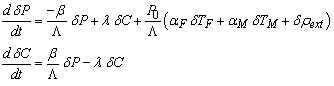(1)

where δP and δC are the changes in reactor thermal power and delayed neutron precursor concentration, respectively; P0 is the steady-state reactor thermal power; β and are λ the delayed neutron fraction and decay constant, respectively; αk represent the reactivity feedback coefficients for fuel (k = F) and moderator (k = M) temperature, δTk; and δρext is an external reactivity input.

When the power level is so low as to make reactivity feedback effects negligible, the point kinetics equations lead to the well-known zero power reactor transfer function of(2)
2.2. Multi-lump Model

In the multi-lump model, the reactor core may be divided in the radial and/or axial directions. Other obvious divisions include the fuel-to-coolant heat transfer in terms of the cladding, gap and the fuel pellet itself. However, because of the relative size difference between these three components, it is the fuel rod length that is generally divided into several sections. Figure 1 shows a block diagram of a typical multi-lump reactor core with N fuel lumps and 2N coolant lumps.

One of the problems brought by the modeling of the heat transfer process is the value of the driving temperature difference (ΔT) between fuel and coolant. The model employed here uses two coolant lumps for each fuel lump with the ΔT for both coolant lumps taken as the temperature difference between the fuel and the average temperature of the first coolant lump. Here, an assumption is made that each coolant lump is well-stirred so that the outlet coolant temperature equals the average coolant temperature in the lump.

The ordinary differential equations for the fuel temperature, TF, are derived from a basic energy balance on the fuel lump from the fission-produced heat gain less the heat transferred to the coolant:(3)

where TF,i is the average temperature of the ith fuel lump; mF is the entire fuel mass in the core; cpF is the fuel specific heat; fi is the fraction of heat generated in the ith fuel lump; τF is the fuel-to-coolant heat transfer time constant; P is the reactor thermal power; and Θi is the average temperature of the ith coolant lump.

In similar fashion, differential equations for the coolant lumps are derived from basic energy balances. For the odd (j = 2i-1) coolant lumps:(4)

For the even (j = 2i) coolant lumps: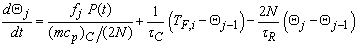(5)

where mC is the coolant mass in the core; cpC is the coolant specific heat; τC is the coolant-to-fuel heat transfer time constant; and τR is the coolant residence time in the core.

Figure 1. Five fuel and ten coolant lumps reactor core model; *only one out of ten coolant and one out of five fuel feedback paths are shown

The frequency response can be ascertained using a matrix formulation. The transfer function can be written as:(6)

where x are the state variables; f = by is the forcing functions vector; and A is the state matrix. For example, the state matrix structure of an isolated core using one fuel and two coolant nodes is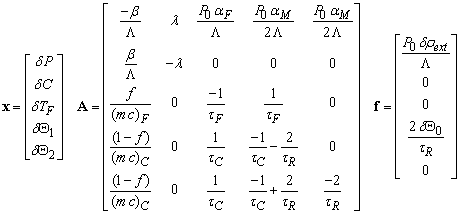(7)

where Θ0 is the core inlet coolant temperature.

Most of the results presented in this study are from a five fuel and ten coolant lumps representation. The block diagram of this model is shown in Figure 1.

2.3. Distributed Parameter Model

The distributed parameter model employs partial differential equations to provide a continuous representation of the spatial dependence of the state variables. In this section, the fuel temperature is first derived, followed by the coolant temperature. Subsequently, the transfer functions in the PWR core are developed. The model is diagrammed in Figure 2.

We assume that the heat transfer through the fuel is one-dimensional in the radial direction with uniform heat generation throughout the reactor core. The partial differential equation describing the heat production and heat conduction through the fuel rod is :(8)

where QF is the heat generation rate per unit volume in the fuel rod; k is the thermal conductivity of the fuel rod; ρF is the fuel density; and cpF is the fuel specific heat. The fuel temperature solution is obtained by Laplace transforming and applying the appropriate boundary conditions including Newton’s law of cooling at the fuel rod surface: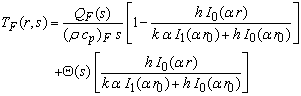(9)

where; h is the surface heat transfer coefficient; and r0 is the radius of the cylindrical fuel rod.

The heat generated is transferred to a flowing coolant. The partial differential equation describing the convection between the fuel element and a single-phase, incompressible coolant with one-dimensional slug flow is(10)

where Θ(z,t) is the coolant temperature; u is the coolant velocity; z is the distance from the core coolant inlet (axial height); p is the heated perimeter of the coolant channel; AC is the cross-sectional flow area of the channel; ρC is the coolant density; cpC is the coolant specific heat; and QC is the volumetric heat generation rate in the coolant. The solution of this relation yields(11)

where(12)

From these results, multiple transfer functions can be extracted. Those of primary interest are explicitly annotated in the overall block diagram of the PWR distributed parameter model in Figure 3, in particular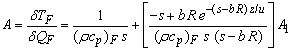(13)(14)(15)(16)(17)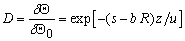(18)

Researchers, such as Gyftopoulos and Smets, used only the feedback from the midplane distributed parameter result to depict core feedback. We, however, have derived analytical expressions that accurately incorporate temperature feedback from across the entire core. Using the block diagram, the overall transfer functions quantifying the change in power to changes in both the reactivity and coolant inlet temperature are, respectively: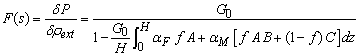(19)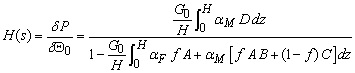(20)

where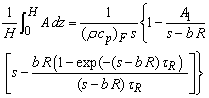(21)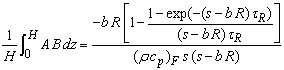(22)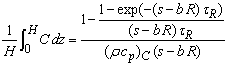(23)(24)

### 3. Simulation and Comparison

In this section, detailed comparisons of the transfer functions obtained from the two models are made using data from a large commercial nuclear power station. The differences between the models are analyzed and explained to illuminate the advantages and disadvantages of the two models. The comparisons made early in this section will be those for which the distributed and multi-lump models are in good agreement. Subsequently, those results that differ significantly are presented to reveal situations that favor one model over the other.

3.1. Power Plant Characteristics

A 3800 MWt PWR is selected for simulation. Table 1 presents those parameters necessary to generate the transfer functions shown hereafter. Uniform heat generation is assumed throughout the reactor core.

3.2. Zero Power Reactor Transfer Function

The zero power transfer function G0, plotted in Figure 4, is a fundamental transfer function that directly impacts the frequency response of other state variables. The graph shows that the frequency response has a zero at approximately 0.0152 Hz and two poles at 0 Hz and around 37.6 Hz, where the former and latter frequencies correspond to λ and λ+β/Λ.

#### Table 1. PWR Operating ParametersDownload asPowerPoint Slide

Veiw figureView current table in a new window

3.3. Power to Reactivity Transfer Function

The overall power to reactivity transfer function (δP/δρext) is graphed in Figure 5. Comparing these curves with that of the zero power transfer function (Figure 4) clearly shows the impact of the fuel and moderator temperature feedback mechanisms. The low frequency gain is reduced to a finite value, while the pole at 37.6 Hz is still present. Notice that the multi-lump model curve is very similar to the distributed parameter model results. We find that the low frequency gain of the distributed parameter model is 41990 MW/ρ, which is close to the multi-lump model value of 40910 MW/ρ. As a gauge of the multi-lump model accuracy, Figure 6 plots the low frequency gain as a function of the number of fuel lumps. As would be expected, greater numbers of lumps begin to better approximate the distributed parameter model, in particular, at about 50 lumps, the multi-lump model reaches the asymptotic value.

3.4. Fuel Temperature to Power Transfer Function

The fuel temperature to power transfer function (δTF/δP) is plotted in Figure 7. The average fuel temperature is obtained at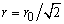using the classic fuel temperature profile of. The fuel temperature response from the distributed parameter model does not vary significantly with axial position since the heat source in this model is assumed uniform along the fuel rod. Without this assumption, the fuel temperature would differ with height. Furthermore, the multi-lump model results are in agreement. The low frequency gain of the distributed parameter model and the multi-lump model are 0.160 °F/MW and 0.164 °F/MW, respectively. The cutoff frequencies for both are 0.0404 Hz, which corresponds to the fuel heat transfer time constant (τF) given in Table 1.

3.5. Fuel Temperature to Reactivity Transfer Function

The fuel temperature frequency response when a change in reactivity is the driving input can be derived from Figure 3:(25)

Figure 8 shows the transfer function in which the low frequency gain is 6550 °F/ρ for the distributed parameter model when z = 6.25 ft (core midplane) and 6411 °F/ρ for the third fuel lump in the multi-lump model. There is greater difference between these two results because the multi-lump model includes feedback from across the reactor core, whereas the distributed parameter result is based purely on feedback at the core midplane. This example is indicative of the need to include complete core feedback in the distributed parameter representation. Even so, both curves match reasonably well. Both curves encounter the first pole at 0.551 Hz, which corresponds to the fuel-to-coolant heat transfer time constant (τF). Then, they both have a break frequency at 37.64 Hz which is caused by the zero power transfer function. After that, it decays from one decade per decade to two decades per decade.

3.6. Coolant Flow Delay

A block diagram of the coolant flow in the multi-lump model is shown as Figure 9, where G2N is the transfer function for each coolant lump. According to the approach taken here, the fuel has been divided into N lumps that each has two corresponding coolant lumps where Θi is the coolant temperature at the exit of the ith coolant node. The transfer function G2N is formulated as: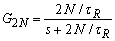(26)

Therefore, the total effect of the multi-lump model is to raise G2N to the power of 2N. By plotting Equation (26) when N equals 1, 5, and 10, and raising it to the power of 2N, the overall effect at the last coolant node (i.e., core exit) can be seen by comparing those curves to that for the distributed parameter model, shown as Figure 10. From the plot, it can be seen that with increasing numbers of lumps, the corner frequency is raised and the asymptotic slope after the break frequency becomes steeper. Hence, the deviation from the distributed parameter model is also increasing with model order. This is very important since logical thought deems that by increasing the number of lumps, the multi-lump model will become more accurate. However, Figure 10 clearly shows that more lumps actually makes this particular result further from the distributed parameter model and leads to very significant error in the estimated frequency response. Hence, a drawback of the multi-lump model is revealed and this effect will propagate to the transfer functions examined in subsequent subsections.

3.7. Coolant Temperature to Heat Production Transfer Function

The overall coolant temperature to heat production transfer function, including heat transfer feedback interactions with the fuel temperature, can be determined as well. For the distributed parameter model,(27)

as shown in Figure 3. Figure 11 plots δΘ/δQC when z = 6.25 ft for the distributed parameter model and the corresponding fifth coolant lump of the multi-lump model as a comparison. The low frequency gains for both are a perfect match at 0.007724 °F/MW.

The effect of the coolant flow delay discussed in Section 3.6 can be observed somewhat in Figure 11 as well. According to Figure 10, the asymptotic slope and break frequency of the multi-lump model differ from those of the distributed parameter model; this effect is slightly exhibited in Figure 11 in the 0.1 to 1 Hz range. In addition, the multi-lump curve breaks upward at ~ 1 Hz. Therefore, it is more accurate to use the distributed parameter model in analyzing this transfer function at higher frequency.

3.8. Coolant Temperature to Reactivity Transfer Function

The transfer function between coolant temperature and reactivity can be derived from Figure 3 as well:(28)

Figure 12 shows that the low frequency gains are 3243 °F/ρ at the core midplane in the distributed model and 3163 °F/ρ for the fifth coolant lump in the multi-lump model. These two models match well at low frequency, but again another case of isolated versus entire core feedback implementation like that in Section 3.5. However, at high frequency, the coolant delay effect shown in Figure 10 is observed in this graph and the multi-lump curve breaks downward at a lower frequency (~ 1 Hz) compared with the distributed model. Therefore, it is more accurate to use the distributed parameter model in analyzing this transfer function above 0.1 Hz.

The high frequency oscillatory behavior is due to the complex exponential term from the coolant flow pure time delay. The frequency of the initial peak is tied to the flow delay time to that axial position, i.e., τz = z/u, which means the oscillation begins at lower frequency, the closer the position is to the core exit. In this case, because of these effects, it may be more accurate to use the distributed parameter model, depending on the frequency interval of interest.

3.9. Coolant Temperature to Fuel Temperature Transfer Function

Next, the coolant temperature to fuel temperature transfer function can be derived from Figure 3: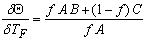(29)

Figure 13 shows the transfer function of coolant temperature to fuel temperature of both models at the core exit. The low frequency gain of the distributed model is 0.0945 °F/°F while it is 0.0949 °F/°F for the multi-lump model. After a turning point at around 1.23 Hz, the gain of the multi-lump model drops to 0.01 °F/°F after the break frequency, while the gain of the distributed model oscillates about 0.01 °F/°F at high frequency. As explained in Section 3.8, the oscillatory behavior at high frequency for the distributed parameter model is also observed in this graph.

3.10. Power to Coolant Inlet Temperature Transfer Function

Figure 14 plots the multi-lump model results and Equation (20) for the distributed parameter model. The low frequency gains of the two models are 29.4 MW/°F for the distributed model and 33.5 MW/°F for the multi-lump model. They both display the break frequency which is caused by the zero power transfer function at 37.63 Hz. At low frequency, these two models match reasonably well. However, the oscillatory behavior of the distributed parameter model presented in Section 3.8 can be seen in this transfer function too. Therefore, it may be more appropriate to use the distributed parameter model in analyzing this transfer function above 0.1 Hz. It is noteworthy that if the number of lumps is increased significantly, to say 100, that the oscillatory behavior starts to manifest itself in the multi-lump response as well.

### 4. Summary

From the comparisons between the multi-lump and the distributed parameter models, the advantages and disadvantages of each are revealed. Based on the methods by which the two models are established, the distributed parameter model is built upon PDEs and it has the ability to offer the transfer function at any location throughout the reactor core. However, the multi-lump parameter model is based on an average depiction. Hence, it can only obtain the value at a certain region (lump) instead of any specific position.

The results presented in this paper have shown that at low frequency (< 0.1 Hz), the multi-lump representation does a remarkable job of approximating the distributed parameter model. It is the portrayal of the pure time delay of coolant flow by first-order lags in the multi-lump model that leads to the most significant differences in the results obtained from the two approaches. In fact, the results (see Figure 10) reveal a finding that is perhaps counter-intuitive, specifically, that larger numbers of lumps can lead to results that increasingly diverge from the distributed parameter case. The simulation results demonstrate that a five fuel-ten coolant lump model provides an accurate depiction of the reactor core behavior.

A contribution here is the inclusion of the fuel and moderator temperature reactivity feedback from the entire core, in the form of analytical formulae. Incorporating whole core feedback becomes even more important when non-uniform heat generation is taken into account.

With the availability of closed-form expressions for the distributed parameter model, their application to reactor parameter identification and analysis are a natural extension to this work. For example, in terms of a noise analysis technique, in , it was necessary to derive the valid regions for ascertaining the moderator temperature reactivity coefficient sign (±) using a multi-lump approach; revisiting that analysis might lead to methodology improvements.

### References

  T. W. Kerlin, “Dynamic analysis and control of pressurized water reactors,” Control and Dynamic Systems, Advances in Theory and Applications, edited by C.T. Leondes, Academic Press, vol. 14, pp. 103-212, 1978.In article  T. W. Kerlin, E. M. Katz, J. G. Thakkar, and J. E. Strange, “Theoretical and experimental dynamic analysis of the H. B. Robinson nuclear plant,” Nuclear Technology, vol. 30, pp. 299-316, Sept. 1976.In article  E. P. Gyftopoulos and H. B. Smets, “Transfer functions of distributed parameter nuclear reactor systems,” Nuclear Science and Engineering, vol. 5, pp. 405-414, 1959.In article  W. Ciechanowicz, “Simulation of the thermal processes in the reactor core based on an exact solution of the thermal diffusion and heat flow equations,” Nuclear Science and Engineering, vol. 13, pp. 75-79, 1962.In article  W. Ciechanowicz, “A comparison of the transfer function of the internal reactor loop for the cases of both distributed and lumped parameters,” Nuclear Science and Engineering, vol. 16, pp. 456-459, 1963.In article  W. Ciechanowicz, On The Simulation of Transfer Functions For a Distributed Parameter System, Kjeller Report KR-59, Institutt for Atomenergi, Kjeller, Norway, 1963.In article  J. A. Thie, Power Reactor Noise, American Nuclear Society, 1981.In article  K. E. Holbert, N. Venkatesh, “Valid ranges for using the cross-power spectral density phase angle for moderator temperature coefficient sign determination,” Nuclear Science and Engineering, vol. 119, no. 3, pp. 203-211, 1995.In article